## Monday, December 30, 2019

### 20/20 Visions

(Click on the comic if you can't see the full image.)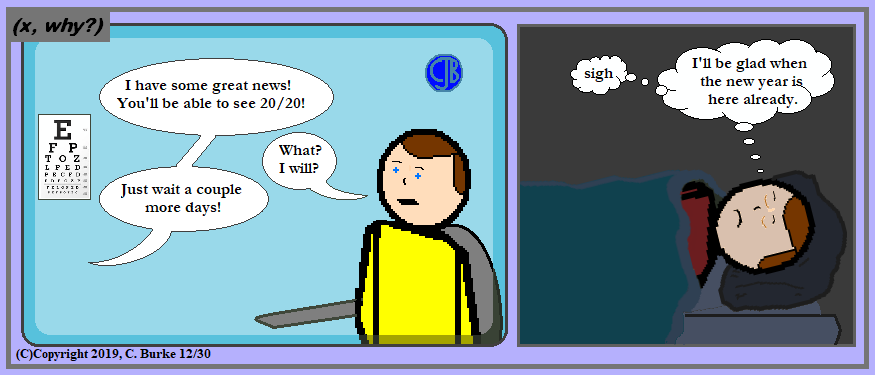(C)Copyright 2019, C. Burke. "AnthroNumerics" is a trademark of Christopher J. Burke and (x, why?).

We're all getting sick of the joke already, right? I'm hearing it in my sleep now!

Come back often for more funny math and geeky comics.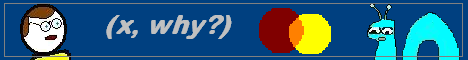## Wednesday, December 25, 2019

### Merry Christmas 2019!

(Click on the comic if you can't see the full image.)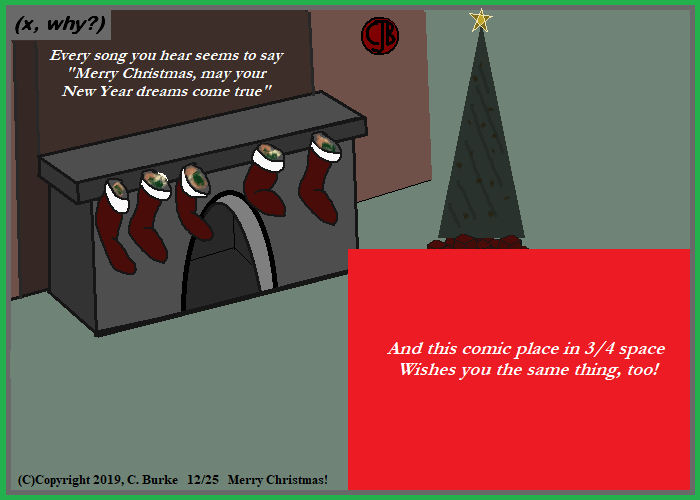(C)Copyright 2019, C. Burke. "AnthroNumerics" is a trademark of Christopher J. Burke and (x, why?).

Merry Christmas, or Merry Christmath!

And, oddly, I had the comic done on the 25th, but then things kept me from posting until late.

Come back often for more funny math and geeky comics.## Tuesday, December 24, 2019

### Happy Christmas Eve 2019!

(Click on the comic if you can't see the full image.)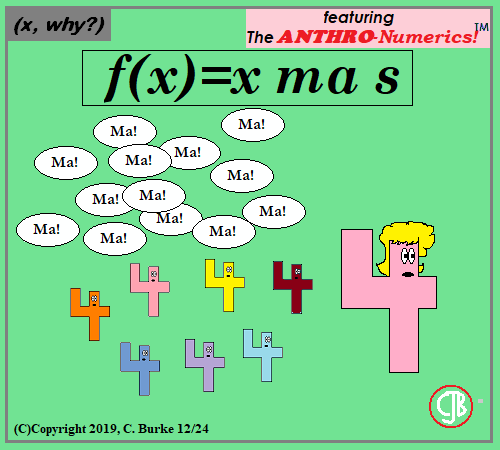(C)Copyright 2019, C. Burke. "AnthroNumerics" is a trademark of Christopher J. Burke and (x, why?).

The number of "ma's" is variable, but generally a large integer that seems to approach infinity.

Exercise for the Reader: Work in an F = ma joke with Fx = xma with an s in it, too.

Come back often for more funny math and geeky comics.## Thursday, December 19, 2019

### Deca-

(Click on the comic if you can't see the full image.)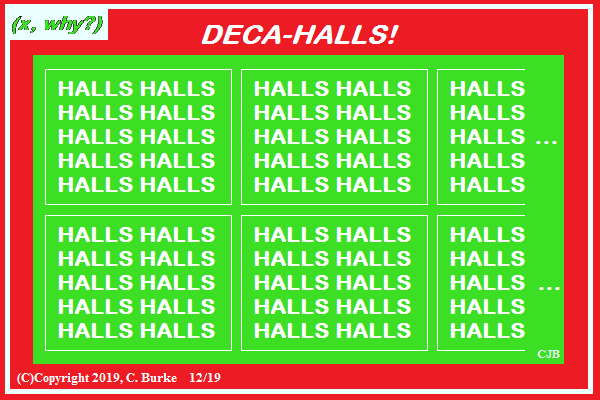(C)Copyright 2019, C. Burke. "AnthroNumerics" is a trademark of Christopher J. Burke and (x, why?).

"Bows" of holly didn't go anywhere, whichever way you pronounce it.

Come back often for more funny math and geeky comics.## Monday, December 16, 2019

### Net Positive

(Click on the comic if you can't see the full image.)(C)Copyright 2019, C. Burke. "AnthroNumerics" is a trademark of Christopher J. Burke and (x, why?).

This is the way words change. Not with a bang, but with an Internet.

Come back often for more funny math and geeky comics.## Saturday, December 14, 2019

### (x, why?) Mini: Theory & Practice

(Click on the comic if you can't see the full image.)(C)Copyright 2019, C. Burke. "AnthroNumerics" is a trademark of Christopher J. Burke and (x, why?).

If Theory is -1, and Practice is 1, then (Theory)(Theory) = Practice, but (Practice)(Theory) does not.

Or maybe there's an absolute power thing going on.

Come back often for more funny math and geeky comics.## Thursday, December 12, 2019

### L4 and L5 Points

(Click on the comic if you can't see the full image.)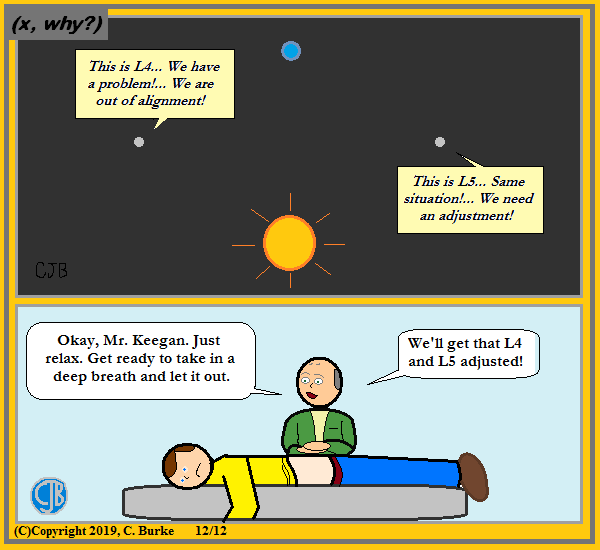(C)Copyright 2019, C. Burke. "AnthroNumerics" is a trademark of Christopher J. Burke and (x, why?).

When I was little, I thought some day I could be part of some L5 Society. And then I turned 40, and I sort of did.

ObMath: For those who are unaware about the L4 and L5 Lagrange points in space, these are two of five points in spaces where the gravitation forces of other bodies (for example, the Sun and the Earth) equal the centrifugal forces felt by the third, smaller object. An object (satellite, space station) in one of these positions would be "parked" in space. They're named about Joseph-Louis Lagrange who wrote about them. What sets L4 and L5 apart from L1, L2, and L3 is that the former two are stable.

To find the location's of Earth's L4 and L5 points, imagine a line segment between the Sun and the Earth. Now imagine that a giant equilateral triangle in space. The L4 point is where the third point of the triangle crosses ahead of the Earth in its orbit. The L5 point similarly would be a point on the orbit behind the Earth.

So there's a giant rhombus is space with points S, L4, E, L5.

Come back often for more funny math and geeky comics.## Wednesday, December 11, 2019

### Books: Math Recreations (Kraitchik) Part 6: Pythagorean Triples... Again

I have more old math books than I'll ever read or need. This is just a fact. I would collect them, and sometimes read through parts of them, but never finish any of them. ...

### Day 6: Pythagorean Triples... Again

If you're a math teacher, and you like numbers, there's no way to avoid Pythagorean Triples, set of three rational numbers which can form a right triangle. I started musing about them when this blog was young -- and blog writing still new to me -- back with this post more than ten years ago. What started that was a curiosity born out of desire to find new numbers to use in math problems. Seriously, almost every triangle was 3-4-5 (or multiple), with the rare exception being 5-12-13 or 8-15-17.

Not that I've ever corrected my main line of reasoning, but I was originally caught up with primitive triples where the hypotenuse was either one or two more than the longer leg. I had Kraitchik's book back then -- there's a page scribbled with notes that's been a bookmark since then. In fact, this is probably where the idea to investigate triples with consecutive legs came from. I hadn't (to my memory) encountered any examples of those before. Surprisingly, but not too much, those pairs were related to the square root of 2. This much I reasoned: the legs are nearly congruent, and if they actually were the same, then the hypotenuse would be radical 2. However, because there are only close to being equal, the hypotenuse would come from an approximation to radical 2. With that in mind, I was able to get through the section on using expanded fractions for the square root of two to get possible triples.

Chapter 4: Arithmatico-Geometrical Questions

I read this chapter (and another book in my collection) back then, but at the time, the notation -- one again -- got to me. In particular, it uses x2 + y2 = z2, instead of the now familiar a2 + b2 = c2. (Ironically, the older version would have made the realization about the formula of a circle so much more obvious, once upon a time.) Moreover, a and b are used for calculating values of x and y. (I've seen m and n used in different sources.) And u and v get toss in for good measure to show the relationship between a and b.

On my own, a long time back, I discovered that instead of looking at the two longest sides, I should have been looking at the two odd numbers in the triples. And once I did that, I saw that the leg was always the hypotenuse minus 2n2. While that didn't give me the triples themselves, it told me that I needed to be looking at pairs of numbers with a difference of 2, 8, 18, etc. (I also came to the conclusion that part of the way I looked at the problem came from the fact that the triples I generally used were relatively small, so I just didn't come across some of them.)

At some point -- as this was me, not any book -- I worked out that for a counting number n, 2n + 1 is the leg of a triple and 2n + 1 + 2n2 is the hypotenuse. The other leg, was the square root of c2 - a2, and I was fine with that. And then I realized b2 = (c - a)(c + a), so I don't have to subtract bigger numbers in my head if I don't want to. And then when I started to work out a table:

 n 2n + 1 2n2 2n + 1 + 2n2 c - a c + a b2 1 3 2 5 2 8 16 2 5 8 13 8 18 144 3 7 18 25 18 32 576 . . .

... I started seeing some of the same numbers showing up. It reminded me of a quiz I gave (special ed class, if I recall correctly) where one student got four out of five problems correct when he used an incorrectly-remembered formula. The four problems he got "right" were all primitive triples. The only that was incorrect was a multiple.

Kraitchik gets into more of the calculation, which I won't repeat here beausee they're likely in so many places on the web (as "helpful" people told me back in 2009), and there, of course, are copyright concerns. After this, there are discussions of Trigonometric Ratios and Heronian Triangles. Thankfully, both are brief and the chapter short. I don't think I would have gotten through something more complex, particularly if it moved in higher dimensions.

Moving on...

I'll likely skip commenting on the next couple of chapters. The chapter on the Calendar was moderately interesting but calculating the day of the week for any day in history isn't something I'm likely to work out with a ruler. There are perpetual calendars and simple apps for that type of thing. The chapter on Probability just isn't all that interesting. There's history, and then there's coin flipping and gambling and Gambler's Ruin. (Side note: it is the one source that says you could win at Roulette, but only because it leaves out the possibility that you will run of money or hit the table limit before you finally win one, which you inevitably will.)

## Tuesday, December 10, 2019

### More Perfect

(Click on the comic if you can't see the full image.)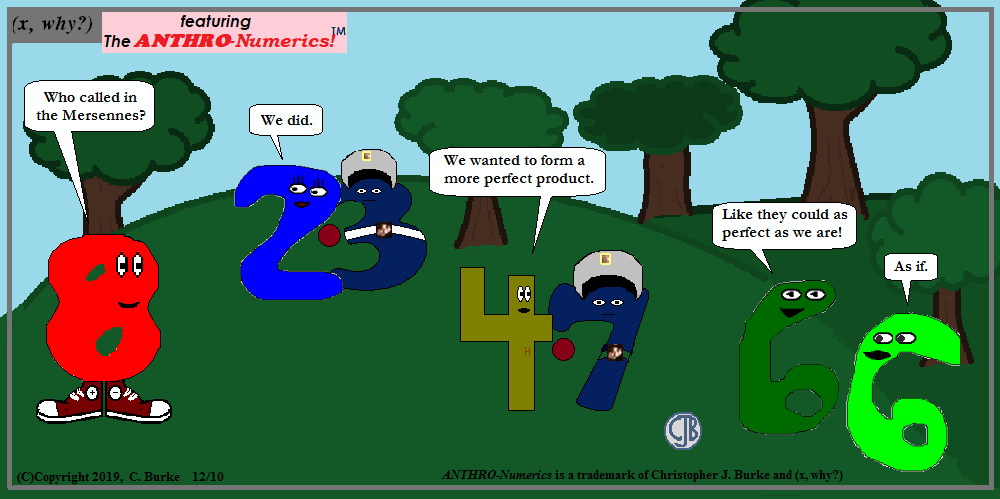(C)Copyright 2019, C. Burke. "AnthroNumerics" is a trademark of Christopher J. Burke and (x, why?).

Tell it to the Mersennes!

I might reuse that line in a future comic. The Mersenne first appeared back in Comic #70 back in 2008. Their uniforms are a little lighter now.

And as discussed in this blog post from a few days ago, every known perfect number is a multiple of a Mersenne prime, and the other number is a power of 2.

Unfortunately for Rudy (8's proper name is Rudolph), 8 is not one of the powers of 2 have creates a perfect number. The form is (2n - 1)(2n - 1). Since 8 = 23, n = 4, but 24 - 1 is not prime, but the product of (22 + 1)(22 - 1). That is to say, that 16 - 1 = 15, which is the product of 5 times 3.

Final note: I was going to state "a more perfect union", in a Constitutional allusion, but I figured folks would tell me that union would imply addition. Or something other than what I intended. So I'll save that for some other time.

Come back often for more funny math and geeky comics.## Saturday, December 07, 2019

### Books: Math Recreations (Kraitchik) Part 5

I have more old math books than I'll ever read or need. This is just a fact. I would collect them, and sometimes read through parts of them, but never finish any of them. ...

### Day 5: Fermat Numbers, Mersenne Primes and Perfect Numbers

Following up to Thursday's entry in this series:

A few final thoughts on this chapter, and then I'll move on.

Fermat Numbers:

I don't think I actually knew what these were. Fermat concluded that numbers in the form 2k + 1, were prime when k was a power of 2. (Read below)
This could also be written as 22**n + 1, where n is a natural number and ** means exponent -- my HTML doesn't allow for exponents of exponents.

The reasoning why the exponents had to be powers of 2 is because k could not have any odd exponents. If k had an odd factor, it could be written as m n, where n was odd.

But we know that we can divide (xm n + 1) by (xm + 1) resulting in xmn - m - xmn - 2m + ... + 1
If that isn't readable, if one factor of the exponent is odd, the expression can be factored (or divided) by one more than x raised to the other factor. (In this case, x = 2.) The result of the division doesn't really matter, as long as we know it's an integer and the original number was composite.

Unfortunately for Fermat, he was wrong about his conclusion, although the first few Fermat Numbers are prime. Euler proved 2^32 + 1 could be factored, and other numbers have been tested now that computers make it easy. (As of the printing of the book, the factorization of many of these numbers is unknown -- only the fact that it isn't prime is known.)

Mersenne Primes and Perfect Numbers

Mersenne primes, I'm familiar with. I even did a comic a long time ago, back in 2008.

Mersenne primes are those that can be written in the form 2n - 1. It doesn't work for all values of n, of course. In fact, they are quite rare, and the search for these primes continues. Since the comic above was created, at least two more have been found.

But we can eliminate many exponents. For example, if n is even, that 2n - 1 can be factored with the Difference of Squares Rule into (2n/2 + 1)(2n/2 - 1). (the exception being 2 itself, because (2 - 1) is a factor of 1.) And if n is composite, then it can be factored similar to the Fermat numbers above, with a similar looking result (except the minus signs will all be addition instead).

So the exponent needs to be prime, and can be written as 2p - 1.

Nothing more needs to be said here. I just wanted the introduction so I could talk about Perfect Numbers. Perfect Numbers are numbers where the sum of all the factors, excluding the number itself, is that number. Example, 1 + 2 + 3 = 6 and 1 + 2 + 4 + 7 + 14 = 28, but 1 + 2 + 3 + 4 + 6 =/= 12.

Again, these are numbers I knew about long, long ago, even before I knew about Mersenne primes, which is why the following formula was such a revelation to me the first time I saw it:

(2n - 1)(2n - 1)

Can we stop and just admire the beauty of this expression.

I learned sometime ago (maybe from reading through this book once before) that Perfect Numbers were always a multiple of a Mersenne prime. That is itself seemed an odd co-incidence. But if you write it out, you see that the other factor is almost the same thing algebraically -- it's the same value of n. The only difference is the placement of the "- 1".

To be sure, this formula does NOT produce perfect numbers for all values of n, BUT as of right now, all known perfect numbers fit that expression. This means that all of the known perfect numbers are, in fact, even. Are there odd perfect numbers? Right now, it is not known, but this formula seems so ... perfect ... that I doubt one will be found.

Stuff like this make math awesome.

## Friday, December 06, 2019

### Upside Down on Your Numbers

(Click on the comic if you can't see the full image.)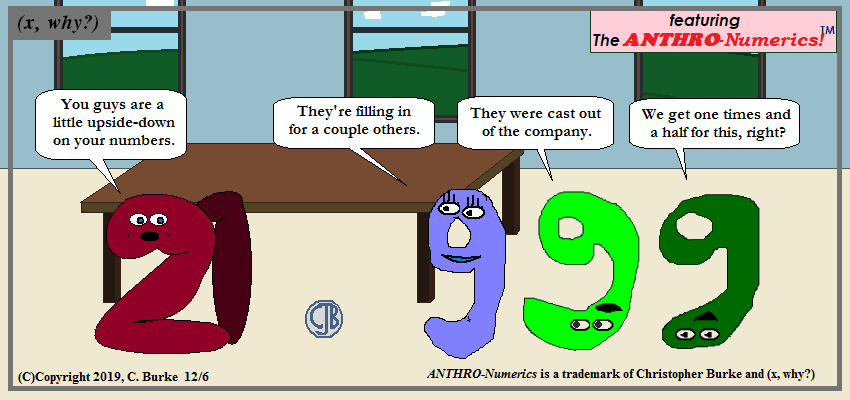(C)Copyright 2019, C. Burke. "AnthroNumerics" is a trademark of Christopher J. Burke and (x, why?).

The numbers have definitely decreased, but working as a 9 is definitely worth one-and-a-half times for a 6.

Not sure what the job is since, in the past, 9 represented the 6's careers. Also a co-incidence that I used Oscar Twenty-one to hire them, which is the sum of 9, 6, and 6.

Come back often for more funny math and geeky comics.## Thursday, December 05, 2019

### Books: Math Recreations (Kraitchik) Part 4

I have more old math books than I'll ever read or need. This is just a fact. I would collect them, and sometimes read through parts of them, but never finish any of them. ...

### Day 4: Final Thoughts about Triangle Numbers and Squares

Continuing from yesterday's very long post:

We've seen images that two triangular numbers make a rectangle, which has dimensions N X (N + 1). If you take a second one and rotate it 90 degrees and place it next to the first, and then repeat this with a third and a fourth, you get a square with the center missing. That center has an area of 1 square unit. Like this:

We know from yesterday that the formula for finding triangular numbers is TN = 1/2 (N2 + N)

That means that 2TN = (N2 + N)

This is important because that middle piece looks familiar, like part of a quadratic expression. (The above image is already a great hint here.)

If I have 8 times a Triangular number, I have

8TN = 8 (1/2) (N2 + N)
8TN = 4 (N2 + N)
8TN = 4N2 + 4N)
At this point, it looks almost like a perfect square, except that there's something missing:
8TN + 1 = 4N2 + 4N)
8TN + 1 = (2N + 1)2

So we can see that the formula 8TN + 1 will always give us a perfect square. Moreover, it will always be an odd square (which makes sense, as it is one more than an even multiple).

The slightly confusing thing (to me) is that, for example, the third triangular number does not lead to the third odd square, but the fourth, because of the + 1 in the formula instead of a - 1.

I guess you can't have everything wrapped in a pretty little bow.

Next up: the book goes into the following topics, but doesn't spend much time on them: Mersenne Numbers and Perfect Numbers, Fermat Numbers, Cyclic Numbers, Automorphic Numbers, Prime Numbers and Multigrades. Then it gets into Cryptarthmetic and other puzzle and games.

There's not much I can add to some of those topics (other than updating data about Mersennes, maybe), and others I've never heard of. So I'll read those and see what I can figure out.

## Wednesday, December 04, 2019

### Books: Math Recreations (Kraitchik) Part 3

I have more old math books than I'll ever read or need. This is just a fact. I would collect them, and sometimes read through parts of them, but never finish any of them. ...

### Day 3: Chapter 3 -- Triangular and Polygonal Numbers

I'm still in Chapter 3, Numerical Pastimes, and it touches on some interesting topics. Among those triangular numbers and polygonal numbers. (These are referred to as "figurate numbers", a term I'm less familiar with, in the text.)

Triangular numbers are of interest because they are the sums of consecutive numbers. And, of course, square numbers are exactly what it says on the tin. After that, pentagonal, hexagonal, etc, are (to me) more curiosities and fun little number puzzles than sums of series of numbers. I'm sure there are uses -- many of which will cause "Aha!" moments at the time -- but they aren't readily obvious as I type this.

The text is quick and dry, but it got my mind thinking. (So that's good, right?)

Personally, rather than go into a bunch of tables, which then derive formulas -- something I could totally do at the end of this blog post, if I'm so inclined -- I wanted to look at these types of numbers visually.

Triangular stack up like a triangle (naturally), so I thought I would build a triangle out of squares, instead of numbers, each with an area of one square unit.

On the right I overlayed a right isosceles triangle, with legs equal to N units (which is this example is 4). The area of the triangle is 1/2 b h, but since the base and the height are both N, the area of the triangle is 1/2 N2. In this example, the triangle has an area of 1/2 (4)2 = 1/2(16) = 8 square units.

But there are little blue bits sticking out of the triangle. There are half triangles that were cut out, and the number of these little triangles is equal to N, because there is one in every row. Collectively, there are N (1/2) (1) (1) = 1/2N, which has to be added to the bigger triangle.

So the total area of the N rows is 1/2 N2 + 1/2 N, or 1/2 (N2 + N), which can also be written as 1/2 (N)(N + 1), or "one-half of the number times the next higher number".

In this example, that becomes 1/2 (4)2 + 1/2 (4) = 8 + 2 = 10, which is the fourth triangle number.

Why would I do all this? BECAUSE I'M HAVING FUN DOING IT!

Yes, I could have copied the triangle, flipped it over, made a rectangle and then noticed that the rectangle was one unit longer than it was wide, and so the rectangle would have an area of (N)(N + 1), and then the triangles would be half as much ... but everyone does that, and you don't need to come here for that, right? (I am, of course, assuming that anyone comes here, other than me, and a couple of friends and relatives who humor me.)

Moving on from triangular numbers to square numbers should show no surprises:

The first time you see this is mind-blowing. I've seen adults caught off-guard by this. When given, as a puzzle, a set of sequences of numbers, and asked to find the next one, the sequence 0, 1, 4, 9, 16, ... is easy, but perhaps not for the reason it should be. There is a pattern: +1, +3, +5, +7 ... and the pattern is +2 to the previous number that was added to the number before that -- it's like two patterns in one. It was taken to the next level ... like to another dimension.

Then point out, "You know that those numbers are all perfect squares, right?" Say what, now? Oh, yeah, they are.

Why should adding odd numbers result in squares? The first time I saw the illustration, it made a lot more sense. Side note: This also makes it easier to find Pythagorean Triples because you are adding something to a perfect square and getting another perfect square. If the number being adding -- for example, 9 or 25 -- is a perfect square, the result is a Pythagorean Triple -- such as 4-3-5 or 12-5-13 because 16 + 9 = 25 and 144 + 25 = 169.

Obviously, the area of the square is length times width, which is N times N, or N2. But the square is made up of two triangles. Looking at it in terms of triangular numbers -- the area would be 2 * (1/2 N2 + 1/2 N) or N2 + N. But in doing this, we counted the diagonal twice, so we need to subtract N from that formula: N2 + N - N or just N2.

Keep with me, I'm going somewhere. I might be lost when I get there, but it will still be somewhere.

Pentagonal numbers are the next extension, and they can be visualized with this:

The problem here is that pentagons don't tessellate, and making a useful, compact figure that maintains this shape is little problematic. On the other hand, I can translate this model into the previous triangle version, as follows:

So the first thing that I noticed: a pentagon can be split into three triangles -- this is how we know that the sum of the interior angles is 540 degrees. And I could split the first pentagonal image into triangles, but it wouldn't helpful. For one thing, they wouldn't be right triangles, so finding the base and height would be impossible. But with this representation, right triangles aren't a problem. I expected three of them. However, instead of getting three triangles with extra half units, like in the first triangular numbers, this image is actually missing those N triangles.

In other words: 3 (1/2 N2) - (1/2 N) or 1/2 (3 N2 - N).
Not exactly what I might have expected when I started. Okay, so my hypothesis was incorrect. That's why we do experiments.

Before moving on, the image looks like a trapezoid, which has an area of 1/2 (b1 + b2) h.
In this example, that becomes (1/2) (N + 2N)N but we have to subtract 1/2N for the missing blocks.
This becomes (1/2) (3N)(N) - 1/2N = 1/2 (3N2) - 1/2n = 1/2 (3N2 - N)

Okay, I realize this is rambling too long. If you ever sit down with me for a conversation, you'll likely feel the same way.

For simplicity, I combined hexagonal, heptagonal and octagonal numbers into one image using the same format. (Obviously, this format would self-destruct in two more iterations.)

Hexagon numbers give us a rectangle. Octagonal numbers look like 3/4 of a square, but are actually more than that -- they're actually a smaller rectangle on top of the bigger rectangle.

Superimposing the right triangle, we can see that we're still losing space.

The hexagonal numbers create a rectangle with a width of N and a length of 2N - 1, as can be seen in the "missing" column. So it has an Area of N(2N - 1) = 2N2 - N. So the hexagonal numbers are double the square numbers but subtracting N.

After this, I ran into a problem. Do I line up my triangles with the missing columns, or should I shift it over. It seemed obvious that I should shift them -- why deal with both "extra" and "missing" pieces in the same problem?

In brief, heptagonal numbers are hexagonal numbers plus a triangle missing space, just as the pentagonal were missing space. This gives us the following:
Area = 2N2 - N + (1/2 N2) - (1/2 N)
Area = (2 1/2)N2 - (1 1/2)N = (1/2)(5N2 - 3N)

Heptagonal looks similar to pentagonal, which differed from triangular because of the sign. But this is where an Aha!! happened. In these three formulas, the first coefficients were 1, 3, and 5. The second coefficients are +1, -1, and -3. Both numbers are sequences. (I know, not surprising -- but I like seeing it visually.)

Moreover, as we can see that octagonal follows hexagonal, which follows square as a pattern: N2, 2N2 - N, 3N2 - 2N, etc. But this is actually the same pattern if we double all of the coefficients and put a leading multiplying of 1/2.

Now that there's only one pattern, I can come up with a single formula for all of this which -- WHICH will likely be the same expression that was presented in the book with little comment or explanation, and which likely caused my brain to freeze up and reach to turn the page. (Actually, the fact that book took it to the third, fourth and nth dimensions caused the brain freeze.)

Yes, notation can be my downfall in mathematics. It's very precise, but decoding it and understanding it can be as bad as translating and understanding any foreign language. And math is definitely a language of its own.

This entry is excessively long at this point, so I'll leave out the table showing the progression. Maybe another day.

However, I can end with the formula. N will which number in the sequence we're looking for, and S will refer to the number of sides in the type of polygon that the sequence is named for.

This means that the Nth S-type number is (1/2)((S-2)N2 - (S-4)N)

And, yes, I do feel better having worked it out myself.

## Tuesday, December 03, 2019

### Number Games

(Click on the comic if you can't see the full image.)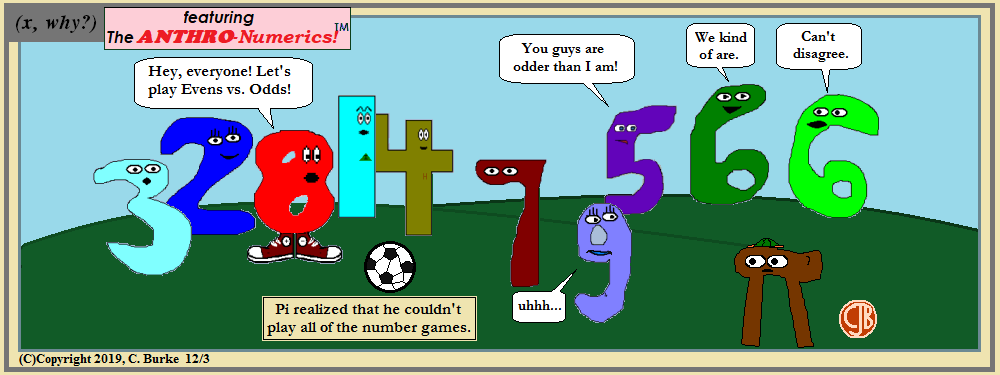(C)Copyright 2019, C. Burke. "AnthroNumerics" is a trademark of Christopher J. Burke and (x, why?).

This is sort of a reindeer games thing. Definitely not a hunger games thing.
Oddly, the Sixes are not odd, weird or irrational, and yet at times they are all three!

Come back often for more funny math and geeky comics.## Monday, December 02, 2019

### Books: Math Recreations (Kraitchik) Part 2

I have more old math books than I'll ever read or need. This is just a fact. I would collect them, and sometimes read through parts of them, but never finish any of them. ...

### Day 2: Chapter 3 -- Numerical Pastimes

Number puzzles can get repetitive quickly if interesting twists aren't added -- but at the same time, those twists shouldn't make the problem impossible to work out on paper.

Some of the problems in this chapter are easy with a calculator instead of paper and ridiculously easy with a spreadsheet or the Internet at hand.

Take a problem like this one:

 Find a four-digit number in the form a a b b that is a perfect square.

Once upon a time, you might have had to start squaring a bunch of two-digit numbers to find the correct answer, or analyze a lot of four-digit numbers to find one that had a rational square root. I could use a spreadsheet in two minutes, and possibly refine it to just show what I wanted with a few minutes more.

But that's no fun.

Knowing that brute force was always a fall-back, we can narrow down the possibilities. For one thing, squaring a number means that the final digit must be 0, 1, 4, 5, or 6. We can eliminate numbers ending in 22, 33, 77, 88 or 99. And 00 isn't possible because no single digit squared becomes a two-digit number consisting of the same digit repeated. (Those pairs are all multiples of 11, a prime number, and not perfect squares.)

Likewise, we can skip 55 because all results from (10N + 5)2 end in 25, not 55.

So we could just square root 1111, 2211, 3311, 4411, etc., until we find it or until we have to move onto 44 and 66. There are only 27 combinations to try.

Don't ask me why, but 11 didn't seem likely to me, so I started with 44 instead. Gut instinct? Pattern recognition that I can't explain?

If you follow that up with, "Okay, same problem but with A A A B B B instead!", it might be less fun to do.

Another problem, with several answers, was along these lines:

 Find a six-digit number ABCDEF, which is a perfect square AND ABC + 1 = DEF.

Again, I took the spreadsheet approach:
• A1: Row() + 100
• B1: =A1 * 1000
• C1: =B1 + A1 + 1
• D1: =C1 ^ .5

Additionally, you could have a conditional in the E column to tell you if you have a whole number in column D to make the visual inspection easier.
Copy this line and paste it down to row 898.

Doing this is brute force because we know that none of the answers ending in 3, 7, 8 or 9 will work. Forty per cent of the spreadsheet we know will fail, but it's quicker to calculate that extraneous information than to just check "good" numbers individually.

One benefit of this approach is that you can alter the columns to explore other ideas, like

• What if DEF is double of ABC? (No need to look beyond 499.)
• What if DEF is half of ABC? (The odd rows will be useless.)

And it isn't a difficult modification to switch to 8 digits.

### SCALES OF NOTATION

A couple of observations to start:
The number 121 is a perfect square in any base, B > 2.
The number 1331 is a perfect cube in any base, B > 3.
The number 14661 is a perfect 4th power in any base, B > 6.

This Pascal triangle allusion is courtesy of the fact that whatever base B is:
B2 + 2 B + 1 = (B + 1)2
B3 + 3 B2 + 3 B + 1 = (B + 1)3
B4 + 4 B3 + 6 B2 + 4 B + 1 = (B + 1)4

Moving on ...

If you wanted to have a set of weights for a balance scale that could measure up to 40 pounds (or kilos, if you metrics prefer), how would you do it?
A quick answer is convert to powers of two, because binary is such an easy solution: 1, 2, 4, 8, 16, and 32. Five weights are needed.
But there is a better solution, using powers of 3: 1, 3, 9 and 27. Only four weights are needed.
This is because you can add to both sides of the balance: if an object is 2 pounds, it will be lighter than 3, but you can put 1 more with the object. So 3 - 1 gives 2.
So an object of 16 pounds can be found if the 27 and 1 are put on one side and the 9 and 3 on the other: 27 + 1 - 9 - 3 = 16.

There was a long proof in the book that gets into "why are you doing that" territory, but I believe it's just showing that 4 weights is the least you can deal with, and is certainly better than 40 1-pound weights.

The amusing part of this section was looking at notation like 1 + x + x2 + x3 + ... + + xn = (xn+1 - 1) / (x - 1)>
I have to remind myself that I just did that in a comic using repeated 9's.

The funny thing is that I didn't think about what that meant for the sum of sequences.
I knew that 1 + 2 + 4 + 8 + 16 = 31, which is 32 - 1, which is (16)(2) - 1, because it's binary, and I have a long history dealing with binary.

But it doesn't naturally occur to me that 1 + 3 + 9 + 27 = ((27)(3) - 1) / (3 - 1) = 80 / 2 = 40. Good to know.

So how much is 5 + 25 + 125 + 625?

## Sunday, December 01, 2019

### Books: Math Recreations (Kraitchik) Part 1

I have more old math books than I'll ever read or need. This is just a fact. I would collect them, and sometimes read through parts of them, but never finish any of them. There are two main reasons: either the math progresses to a point where I started losing interest in college, maybe starts using odd notation I can't recall; or it's more of a books of games, puzzles and logic, which after a while start to feel repetitive or like homework, possibly delving into areas or games I'm not particularly interested in.

In the case of notation, there are times when I think books should give an equation. Then rewrite it in English. Then explain in English what the previous sentence meant. And maybe explain why we're even doing this. The funny thing about that last one is that they usually are explaining why they're doing it -- just not in a way that makes a whole lot of sense to me by that point.

Anyway, I've decided that I'm going to start skimming through these books, following along as I can, and solving what I feel like solving, while nodding, yeah, I can do that, without actually solving other things. And as I move on to the next book, I'll donate, or recycle, the one I just finished. I'm not sure where exactly I could donate them -- most of the ones I have were removed from library circulation (either the public library or a public school library).

The first one I picked up, with its patterned green cover, is Mathematical Recreations by Maurice Kraitchick, Dover Publications, Inc, 1942. It was removed from the William E. Grady Technical Vocational High School Library sometime before 2010. ("In the late 2000s" did not seem like the correct phrase.) While there is no entry for it in my reading blog (which has a multi-year gap when I stopped posting), it did have a sheet of paper where I wrote calculations about Pythagorean Triples which ended up in blog posts over ten years ago.

The first chapter is MATHEMATICS WITHOUT NUMBERS and is the situational kind of logic problems that I've seen before whether I understood them or not at the time.

There are the Always-Lies/Always-Tells-the-Truth problems. The smart people/philosophers who can guess if there face is marked, what card is one their head, what color is on their back. Spoiler alert: they're all the same, but the reasoning was a little convoluted first time I saw it way back when. If they were really smart, they'd say, "I know the answer to this because it's always the answer to this problem!" That would similar to the student who writes: "the statement is true because every proof on a state exam is provable and true".

Sample Puzzle

A typical Three-Smart-Person problem is the three are sleeping and someone comes in and marks their faces with black marker. The each wake up at the same time and start laughing at the others and then stop laughing when they figure out that their own face must be blackened. How did they figure this out?

Again, it relies on the fact that they are smart, and they know that the others are smart.

A sees that B and C are laughing. A knows since B is laughing, he thinks that his face is clean. B can see C laughing as well. Now follow me here.

If A's face was clean, and B could see that A's face was clean, and B thought his own face clean, then B would be astonished that C was laughing because C would have nothing to laugh about. But since B is not puzzled by C's reaction, B must know that A's face is dirty. (And the other two think similarly.)

Me? I think in that situation, I'd be pointing at the other two as well as laughing, and then realize, why would someone spare me, unless they wanted to frame me for doing it.

Another puzzle of the situational kind involves a man who cannot beat two seasoned chess players even with a piece advantage and playing white, but who can beat his daughter, nevertheless watches as his daughter plays both players simultaneously with no advantages, allowing one to be white and the other black, and performing better than her father did. How does this happen?

It's the kind of thing where you have to think outside the box a bit. Basically, the young girl doesn't play at all. She mimics each player's moves on the other board, thus insuring that she will either win one game and lose the other, or that both games will be a draw. In reality, the seasoned players might find this amusing at all, but they'd soon quit or just leave out the middle person.

Chapter 2: Ancient and Curious Problems

So I moved onto Chapter 2 pretty quickly, and it has the advantage (for this blog) that parts of it are not under copyright because they come from other sources, namely Chuquet (1484) and Clavius (1608). As a math teacher, but not a mathematician or math historian, I'm not exactly familiar with either of these. Shame on me, I know. Also, there are problems from the Greek Anthology, a collection of epigrams (short poems).

I won't put all 50+ of them here -- and I might not even work them all out, even with the answers immediately below the problem (no back of the book!) -- but here are a few puzzles for my few readers to work on.

PUZZLES

1. A man spends 1/2 of his money and loses 2/3 of the reminder. He then has 12 pieces. How much money did he have at first? (Chuquet)

2. A bolt of cloth is colored as follows: 1/3 and 1/4 of it are black, and the remaining 8 yards are gray. How long is the bolt? (Chuquet)

3. A merchant visited three fairs. At the first, he doubled his money and spent \$30, at the second he tripled his money and spent \$54, at the third he quadrupled his money and spent \$72, and then had \$48 left. How much did he start with? (Chuquet)

As you can see at this point, (1) and (3) are similar in that you have to work backward for the final answer, although (3) is a bit more involved. I have given similar problems in Algebra class to see how students approach problem solving.

I know how to approach these, so more problems of this kind quickly become less interesting in the book (particularly since the answer is printed below.)

12. "Best of clocks, how much of the day is past?" "There remain twice two-thirds of what is gone" (xiv. 6)
Assuming a 24-hour clock, h has passed and 2(2/3)h remains, so h + 4/3h = 24, so 7/3h = 24, and h = (24)(3/7) = 72/7 = 10 2/7 hours have passed.
Okay, I'll do that once, but stop making it seem like homework.

I'll leave off with one last example, because I'm not sure exactly how much is fair use here, even with paraphrasing:
Lewis: Give me \$10 and I'll have three times as much as you.
Carol: And if I get the same from you, I'll have five times as much as you.
How much do Lewis and Carol have? (not the original names)

And, once again, the very next problem is almost the same thing. I already know the steps. Am I going to go through them again?
Yeah, probably. And then I'll move on to Chapter 3.

### BONUS PROBLEM

As posed by Frederick II to Fibonacci (Leonardo di Pisa) in 1225: Find a perfect square that remains a perfect square when increased or decreased by 5.
Luckily (for me), the explanation is on the following page. Since 4 + 5 = 9, but 9 + 5 is not a perfect square, I'm guessing that there are "crazy" numbers involved! (i.e. fractions).